Lines and Circles

## Topics from Lines and Circles

List of animations posted on this page.(Click the text to watch animation.)

### 1. Conics as Envelopes of Lines

#### Conics as envelope of lines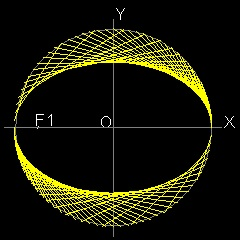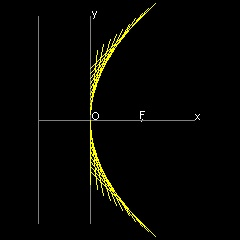#### Conics by drafting triangle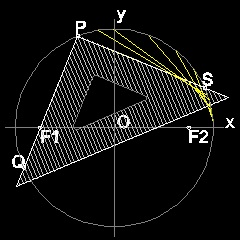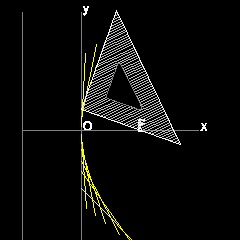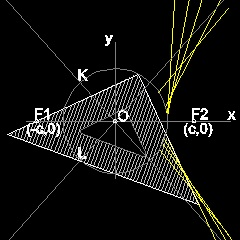#### Conics by paper folding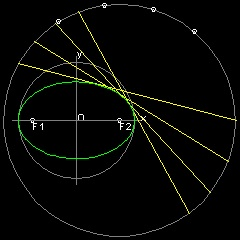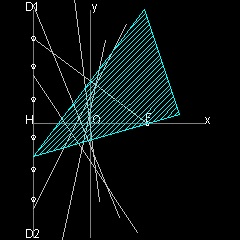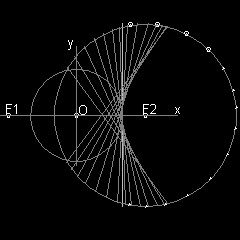For details, go to Ellipse ,Parabola and Hyperbola .

### 2. Cycloid, Epi & Hypo-cycloid as Envelopes of Lines

#### Cycloid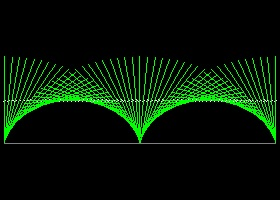-------See cycloid_by_line envelope #0 -------See cycloid_by_line envelope #1 -------See cycloid_by_line envelope #2

For details, go to Cycloid,Epi & Hypo-cycloid as Envelopes of Lines

#### Epi-cycloid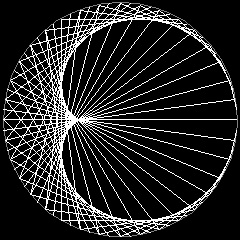For details, go to Epicycloid

#### Hypo-cycloid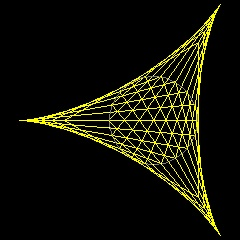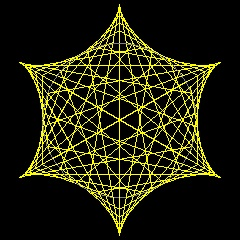For details, go to Hypocycloid

### 1. Conics by Envelope

The Conics (Ellipse, Parabola & Hyperbola) was invented by a Greek mathematician, Menaechmus (about 380 BC - about 320 BC) , tutor to Alexander the Great , in an attempt to solve "Delian Problem or Duplicating the Cube", which is one of the "Three Famous Problems" in Greek mathematics.
Further studies were done by Apollonius of Perga (about 262 BC - about 190 BC).
There are many excellent references on this subject, and the ones the author could purchase via internet are listed in the reference section at the bottom of this page.

#### 1.1 Ellipse

##### Envelope of lines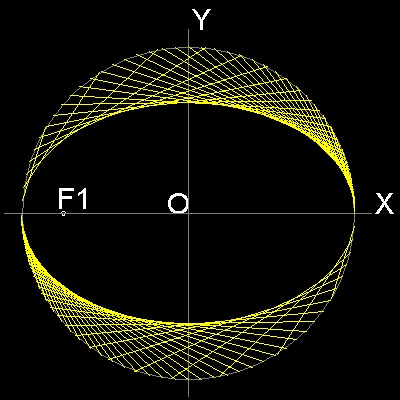************* ellipse_manual_2.dwg ************* **************** ellipse_auto.dwg ****************

You can see the process in animation.

To create this drawing and animation:
Then from command line, type ellipse_auto
To create ellipse_manual_2.dwg, type ellipse_manual_2 in command line window.

##### Description of ellipse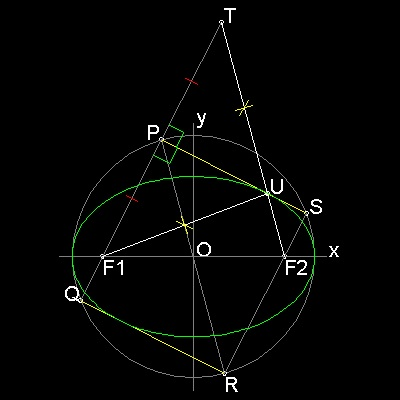```Draw a circle with radius = a.
Select a point F1 with distance c from O.
From a point P on the circle ,draw a line through F1 to Q on the circle.
Draw a line PS perpendicualr to PQ.
Make a rectangle PSRQ. It cuts x-asix at F2.
Extend line F1P and make point T such taht F1P = PT
Connect T-F2, This intersects PS at U.
Since PQ is parallel to SR, triangles OPF1 is congruent to trianlge ORF2.
Since trainlges UPF1 and UPT are congruent, F1U = UT.

Therefore F2 is (c 0), and UF1 + UF2 = UF2 + UT = PR = 2a (constant)
So the point U is on the ellipse with foci F1,F2.
and also tangent to the curve at U.
Envelope of many lines drawn like "PS" ,"QR" will
make an ellipse as shown above.
```
To create this drawing :
Then from command line, type ellipse_model
*************** ellipse_model.dwg ***************
##### Using a drafting triangle
Using the property that points P,Q,& S are on the circle,and angle SPQ is a right angle, line PS can be drawn by using a drafting triangle as follows.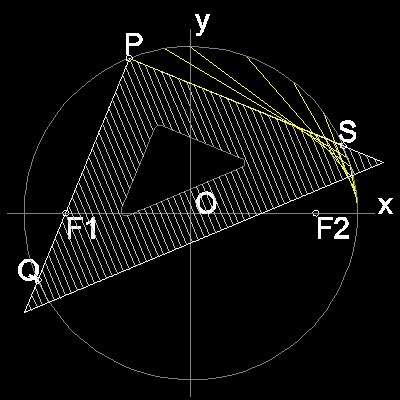You can see the process in animation.

To create this drawing and animation:
Then from command line, type ellipse_by_triangle
************ ellipse_by_triangle.dwg ************

##### Folding a cicular paper
It was shown that point T is on the circle with its center at F2 and radius 2a.
When that circle is drawn , it becomes clear that line PS can also be a part of the line VW,
which is created as a crease line when a circular paper is folded so that point T falls on point F1.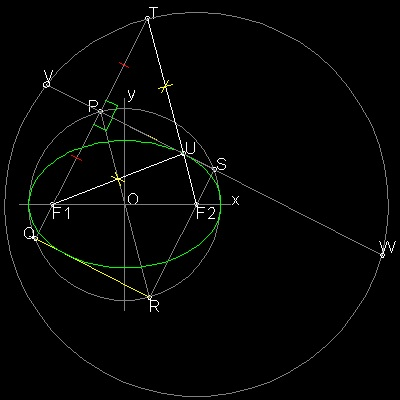To create this drawing :
Then from command line, type ellipse_model
Answer "Yes" to "Want to continue ?" prompt.
************** ellipse_model_2.dwg **************

paper folding result: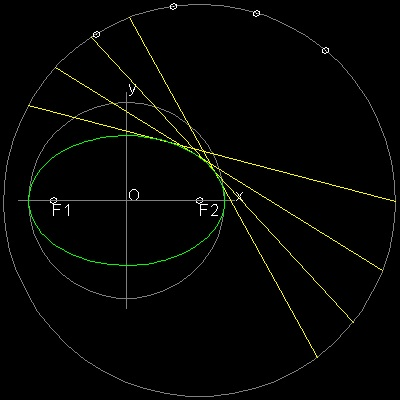You can see the process in animation.

To create this drawing and animation:
Then from command line, type ellipse_by_folding
************* ellipse_by_folding.dwg *************

#### 1.2 Parabola

##### Envelope of lines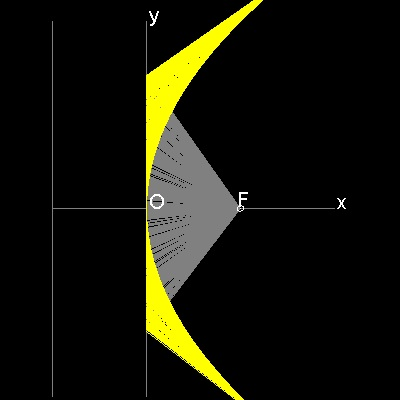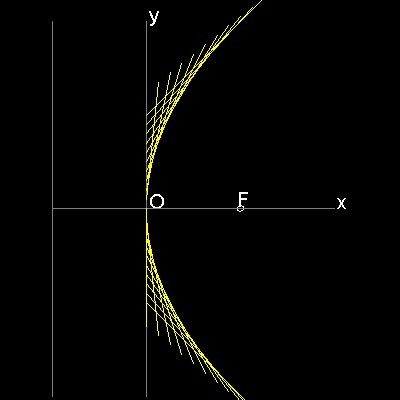************* parabola_manual_2.dwg ************* **************** parabola_line.dwg ****************

You can see the process in animation.

To create this drawing and animation:
Then from command line, type parabola_auto
To create ellipse_manual_2.dwg, type ellipse_manual_2 in command line window.

##### Description of parabola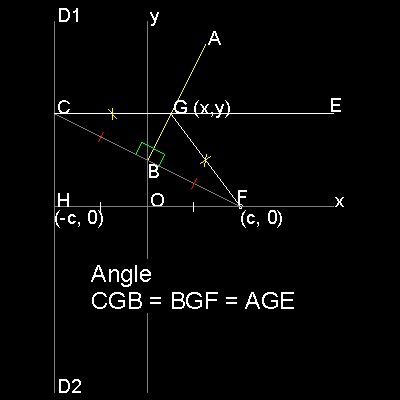```F is a Focus (c, 0), and line D1 D2 is parallel to y axis
with distance c from the origin O.(called Directrix)
B is a point on y-axis. FB intersects with directrix at C.
Line AB is perpendicular to line FC at B.
Draw a line from C parallel to x-axis, and this intersects AB at G.
Then GC = GF -->definition of parabola
Furthermore, angle CGB = BGF = AGE.-->angle bisector of EGF is normal to AB
So point G is not only a point on the parabola,but line AB touches this parabola
at point G.
Consequently envelopes of lines like AB will form a parabola.
```

To create this drawing :
Then from command line, type parabola_model
*************** parabola_model.dwg ***************
##### Using a triangle

Using the property that points B is on y-axis,and angle ABF is a right angle, line AB can be drawn by using a drafting triangle as follows.You can see the process in animation.

To create this drawing and animation:
Then from command line, type parabola_by_triangle
************ parabola_by_triangle.dwg ************

##### Folding a paper
In the model drawing below, point C on directrix and Focus F are symmetric with respect to line AB.
So line AB is created by a crease on the paper when a paper is folded such that a point on directrix falls on the Focus F.
Note here that directrix D1 D2 is considered as an arc of a circle with its center at infinity on x-axis.To create this drawing :
Then from command line, type parabola_model
************** parabola_model_2.dwg **************

Parabola by folding a paper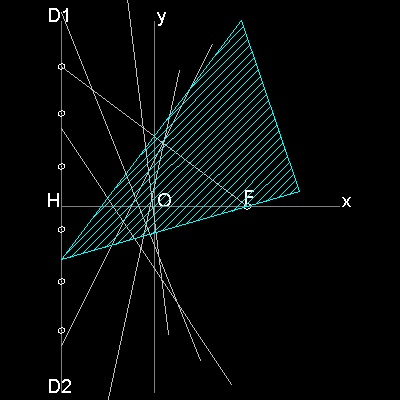You can see the process in animation.

To create this drawing and animation:
Then from command line, type parabola_by_folding
************* parabola_by_folding.dwg *************

#### 1.3 Hyperbola

##### Envelope of lines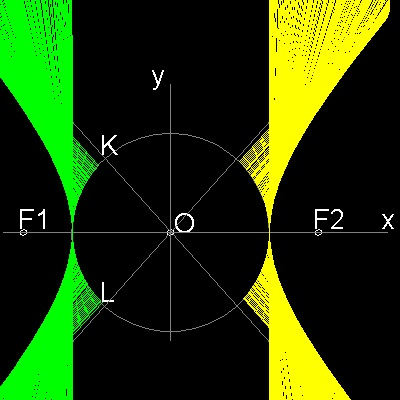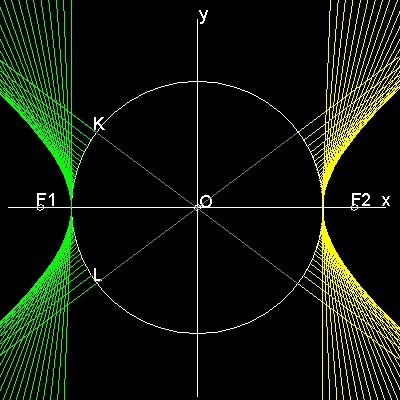*********** hyperbola_manual_2.dwg *********** ************** hyperbola_auto.dwg **************

You can see the process in animation.

To create this drawing and animation:
Then from command line, type hyperbola_auto
To create hyperbola_manual_2.dwg, type hyperbola_manual_2 in command line window.

##### Description of hyperbola```Draw a circle with radius = a.
Select  points F1,F2 outside of this circle with distance c from O.
From a point P on the circle ,draw a line through F1 to Q on the circle.
Draw  lines PB and RA perpendicualr to PQ.They intersect the circle at R & S.
PSRQ is a rectangle. Extention of RS goes through F2 due to symmetry.
Extend line F1P and make point T such that F1P = PT
Connect T-F2, This intersects PS at U.

Since triangles UPF1 and UPT are congruent, F1U = UT
Therefore  UF1 - UF2 = UT - UF2 = F2T = 2a (constant).
So the point U is on the hyperbola with foci F1,F2.
Furthermore since angles PUF1 = PUT = BUV, line
BP is a tangent line to this hyperbola at U.
```

To create this drawing :
Then from command line, type hyperbola_model
************* hyperbola_model.dwg *************
##### Using a triangle

Using the property that points P,Q,& S are on the circle,and angle SPQ is a right angle, line PS can be drawn by using a drafting triangle as follows.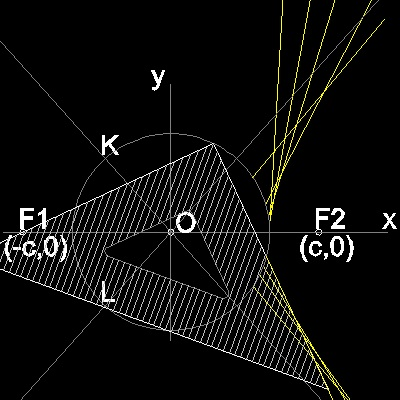You can see the process in animation.

To create this drawing and animation:
Then from command line, type hyperbola_by_triangle
************ hyperbola_by_triangle.dwg ************

##### Folding a cicular paper
It was shown that point T is on the circle with its center at F2 and radius 2a.
When that circle is drawn , it becomes clear that line PS can also be a part of the line VW,
which is created as a crease line when a circular paper is folded so that point T falls on point F1.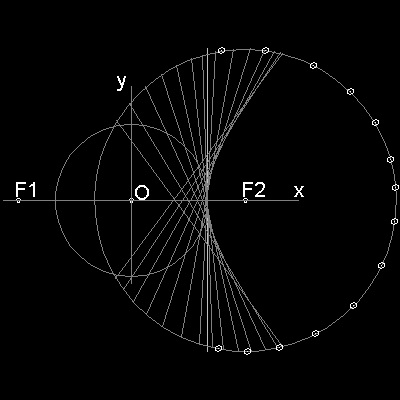You can see the process in animation.

To create this drawing and animation:
Then from command line, type hyperbola_by_folding
********** hyperbola_by_folding.dwg **********

### 2. Cycloid, Epi & Hypo-cycloid as Envelopes of Lines

#### 2.1 Cycloid

##### Envelope of lines******************* cycloid_by_line_envelope.dwg ************************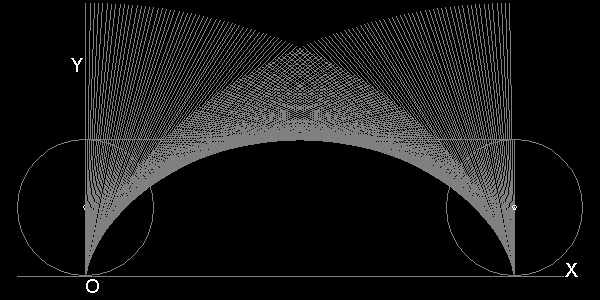******************* cycloid_by_line_envelope_2.dwg *********************

You can see the process in animation.

To create this drawing and animation:
Then from command line, type draw_cycloid_by_line
1-st example: no. of division = 20
2-nd example: no. of division = 200, and layer2 (yellow colored nodes) is hidden.

##### Description of cycloid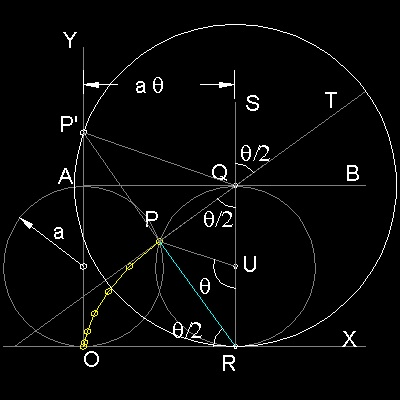```Point P on a circle with radius "a" is at point O initially.
After this circle rolls along X-axis by θ,
top, center and bottom of this circle are points Q,R & U respectively.
Since R is the instantaneous center of rotation, ∠RPQ is a right angle,
and line PT is tangent to the curve formed by locus of P.
∠PUR = θ, and ∠PRO = ∠PQR = ∠SQT = θ/2
Length of OR = a θ.
This yields the following parametric equation for cycloid.
x = a(θ - sin θ)
y = 2a(1 - cosθ)
Envelope of many lines like "PT" , will
make a cycloid as shown above.
This can be accomplished by either of the two ways:
(1)Draw a horizontal line AB with lenght 2π, and divide in n equal lengths.
Draw a line at each point with an angle incremented by 2π/n.
(2)Draw a circle with radius 2a, and draw a diameter vertical initially at O.
Roll this big circle along X a-xis, then the envelope of this diameter
forms cycloid. The reason is that when the big circle comes to R,
∠RQP' = θ and PT is a part of the diameter.
```

*************** cycloid_model.dwg ***************
To create this drawing :
Then from command line, type draw_cycloid_model
Use 20 division and stop execution at the 7-th step. Modify the drawing .

#### 2.2 Epi-cycloid

The Epicycloid is generated by a point of a circle rolling externally upon a fixed circle.
##### Examples of Epicycloid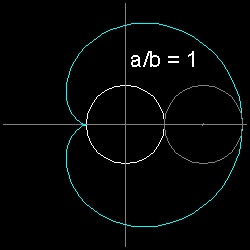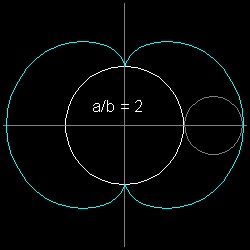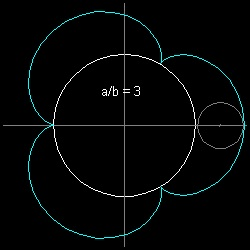** epicycloid_cardioid.dwg ** epicycloid_nephroid.dwg ** epicycloid_cremona.dwg
** draw cardioid-animation ** draw nephroid-animation ** draw cremona-animation

##### Description of Epicycloid```T.B.A.
```

************* epicycloid_model.dwg *************
To create this drawing :
Then from command line, type draw_cycloid_model
Use 20 division and stop execution at the 7-th step. Modify the drawing .
##### Epicycloid by String Art (Curve Stitching)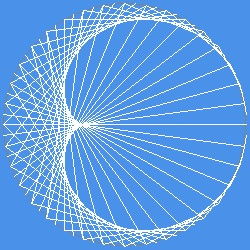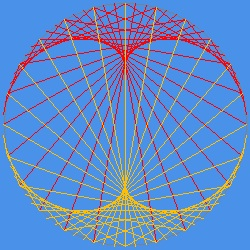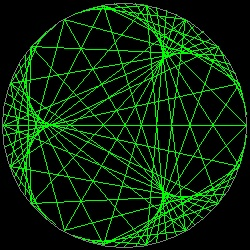**** string_cardioid.dwg**** **** string_nephroid.dwg**** **** string_cremona.dwg****
** draw cardioid-animation ** draw nephroid-animation ** draw cremona-animation

#### 2.3 Hypo-cycloid

Under construction

#### References

Go to   Fun_Math Content Table

All questions/complaints/suggestions should be sent to Takaya Iwamoto

Last Updated Aug 6-th, 2007